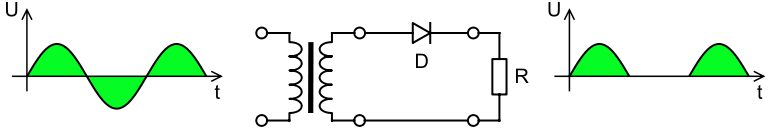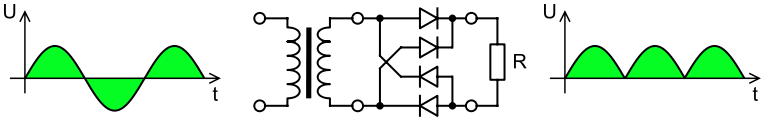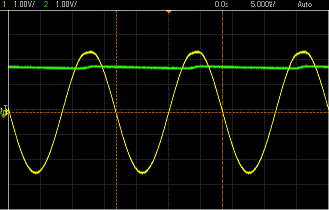HOME >> RELEASE >>

AC Power adapters convert a higher-voltage alternating current to a lower-voltage direct current for use with devices that require a relatively constant voltage (within a tolerance). Direct current is also required to recharge batteries, making AC adapters essential for electronic devices like laptops (see Figure 1) and cell phones. Older AC adapters waste quite a bit of electricity in the form of standby power, but recent regulations and subsequent innovations have reduced the amount of electricity wasted considerably. See Standby power for more detailed information.

## How it works

A simple AC adapter consists of a transformer, a rectifier, and an electronic filter. The transformer initially converts a relatively high-voltage alternating current that is supplied by an electrical outlet to a lower voltage suitable for the device being powered. A rectifier then converts alternating current into direct current. There are two separate types of rectifiers: the half-wave rectifier and the full-wave rectifier.

### Half-wave rectifier

The half-wave rectifier effectively blocks current flow in one direction through the use of a diode (see figure 2). This creates a pulsating direct current. Due to half of the current being blocked, much of the supplied energy is lost, and the mean voltage of half-wave rectified power is lower than that of full-wave rectified power.Figure 2. A half-wave rectifier. The arrow-shaped symbol at the top of the electric circuit is a diode that only allows electron flow from right to left across the diode. The graph on the left is the input voltage and the graph on the right is the output voltage.

### Full-wave rectifier

There are several types of full-wave rectifiers. Rather than blocking the electric current in one direction, these rectifiers flip its direction so that a constant pulsing direct current is the output. A common type of full-wave rectifier is the full-wave bridge rectifier (See Figure 3).Figure 3. A full-wave bridge rectifier. The graph on the left is the input voltage and the graph on the right is the output voltage.

### SmoothingFigure 4. The output voltage (green) of a half-wave rectifier and smoothing capacitor from the input AC voltage (yellow). Note the ripples in the output voltage.

As seen in Figures 2 and 3, the output voltage of the two rectifiers still varies greatly. However, a constant voltage is required for most devices that operate on direct current. A smoothing circuit or capacitor is used to achieve this. At its simplest, a circuit component known as a reservoir capacitor or smoothing capacitor is placed at the output of the rectifier. Essentially it releases stored energy when no voltage is being output from the rectifier. This creates a fairly steady voltage. However, some ripples from the AC voltage remain (See Figure 4).